Refer to our Texas Go Math Grade 3 Answer Key Pdf to score good marks in the exams. Test yourself by practicing the problems from Texas Go Math Grade 3 Module 4 Assessment Answer Key.

Vocabulary

Choose the best term from the box.

Question 1.
________ are numbers that are easy to compute. (p. 107)
Explanation:
compatible numbers are the numbers that are easy to add, subtract, multiply, or divide mentally. Compatible numbers are close in value to the actual numbers that make estimating the answer and computing problems easier.

Question 2.
The ____________ states that you can group addends in different ways and still get the same sum. (p. 113)
Explanation:
Property of Addition states that the way addends are grouped does not change the sum. Property of Addition states that the numbers can be added in any order and the sum will be the same.

Question 3.
A number close to an exact number is called an ___. (p.107)
Explanation:
A number close to an exact number is called an estimate

Concepts and Skills

Use rounding or compatible numbers to estimate the sum. TEKS 3.4.B

Question 4.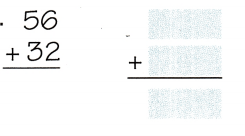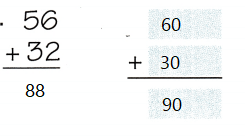Explanation:
Estimation is 90

Question 5.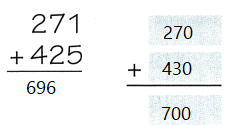Explanation:
Estimation is 700

Question 6.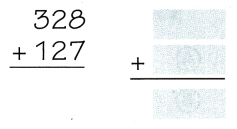Explanation:
Estimation is 90

Use properties to add. TEKS 3.4.A

Question 7.
46 + 14 = ___
Explanation:
40 + 6 + 14 = 60
The associative property of addition says that no matter
how a set of three or more numbers are grouped together,
the sum remains the same.

Question 8.
39 + 243 = ____
Explanation:
39 + 200 + 43 = 282
The associative property of addition says that no matter
how a set of three or more numbers are grouped together,
the sum remains the same.

Question 9.
326 + 402 = ___
Explanation:
326 + 400 + 2 = 728
The associative property of addition says that no matter
how a set of three or more numbers are grouped together,
the sum remains the same.

Estimate. Then find the sum. TEKS 3.4.A, 3.4.B

Question 10.
Estimate: ______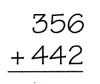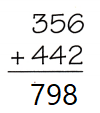Explanation:
Estimate: 700

Question 11.
Estimate: ____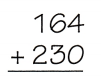Explanation:
Estimate: 300

Question 12.
Estimate: ____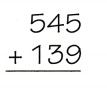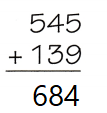Explanation:
Estimate: 600

Question 13.
Estimate: ____Explanation:
Estimate: 900

Question 14.
Nancy planted 48 roses and 39 tulips. Which is the best estimate for the number of flowers she planted?
Record your answer and fill in the bubbles on the grid. Be sure to use the correct place value. TEKS 3.4.B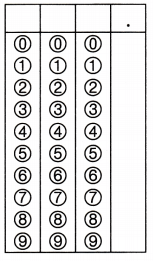Explanation:
The best estimation of  48 + 39 = 50 + 40 = 90

Fill in the bubble for the correct answer choice.

Question 15.
Jacob has some money in his bank. The money is shown below. How much money does he have in his bank? TEKS 3.4.C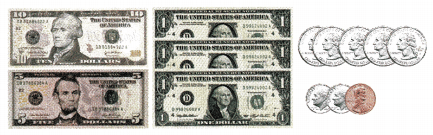(A) $18.23 (B)$20.48
(C) $19.28 (D)$19.46
Explanation:
Jacob has \$19.46

Question 16.
There are 294 boys and 332 girls in the Hill School. How many students are in the school? TEKS 3.4.A
(A) 526
(B) 637
(C) 626
(D) 172
Explanation:
626 students are in the school.

Question 17.
On Monday, 76 students played soccer. On Tuesday, 62 students played soccer. On Wednesday, 68 students played soccer. How many students played soccer on those three days? TEKS 3.4.A
(A) 207
(B) 196
(C) 206
(D) 216